Chapter 10

As we know, the velocity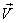, the mass m, and the momentum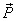has the follows relationship: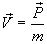(10.1)

Let us make the assumption that the above relationship is also valid within the electromagnetic field. As we know for the electromagnetic field, the momentum density is: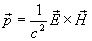(10.2)

Per our assumption, the mass origin is from the electromagnetic field energy, so the mass density inside the electromagnetic field is:(10.3)

In which u is the electromagnetic field energy density: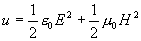(10.4)

Thus, the velocity of electromagnetic field is as follows: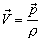(10.5)

Thus: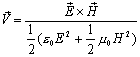(10.6)

As we know: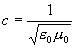Thus:(10.7)

Equation (10.7) is the electromagnetic field velocity equation.

From the equation (10.7), we found out that the value of velocityis always less than the speed of light c, when and only when both of the follows two conditions are satisfied, the velocity will equal to speed of light, which are: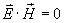(10.8)

And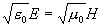(10.9)

Thus:(10.10)

Combine the equations (4.2) and (5.2) into (10.7), then we can get the velocity of electromagnetic field inside the electron as follows: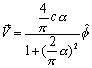(10.11)

In which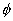is the azimuthal angle.

From the above equation, we find out that inside of the electron, the velocity is around the azimuthal direction, and that the speed inside of the electron has constant value.

As we know: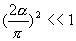Thus: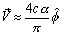(10.12)

Reference

Wilhelm Fushchych

Journal of Nonlinear Mathematical Physics 1998, V.5, N 2, 159–161.

A note on magnetic monopoles and the one dimensional MHD Riemann problem
Paul J. Dellar
Journal of Computational Physics 172, 392–398 (2001)

Conservation of Circulation in Magnetohydrodynamics
Jacob D. Bekenstein, Asaf Oron
Phys.Rev. E62 (2000) 5594-5602

James M. Gelb, Kaundinya S. Gopinath, Dallas C. Kennedy

Int.J.Mod.Phys. D8 (1999) 229

Hamilton's principle for electromagnetic fluids
H. A. Haus and P. L. Penfield
Phys. Fluids 9, 1195-1204, Feb. 1966.

Towards an Exact Mechanical Analogy of Particles and Fields

Valery P.Dmitriyev

Nuov.Cim. 111A, N5, pp.501-511 (1998)

Valery P. Dmitriyev

Apeiron, vol. 7, no 3-4, pp. 173-183 (2000)

Spinning magnets and Jehle’s model of the electron
D.F. Bartlett et al
Physical Review D, 16 (1977).

Spinning magnets and relativity. Jehle vs. Bartlett
Guala-Valverde
Physica Scripta, The Royal Swedish Academy of Sciences, 66, (2002).